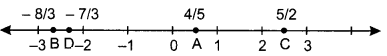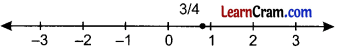# DAV Class 7 Maths Chapter 1 Worksheet 5 Solutions

The DAV Class 7 Maths Book Solutions Pdf and DAV Class 7 Maths Chapter 1 Worksheet 5 Solutions of Rational Numbers offer comprehensive answers to textbook questions.

## DAV Class 7 Maths Ch 1 WS 5 Solutions

Question 1.
State whether the following statements are true: If not, justify your answer.
(i) On a number line, all the numbers to the right of zero are positive.
True

(ii) Rational number $$\frac{-7}{-19}$$ lies to the left of 0 on the number line.
False, as $$\frac{-7}{-19}=\frac{7}{19}$$ is a positive rational number which always lies on right of the zero.

(iii) On a number line, numbers become progressively larger as we move away from zero.
True

(iv) Rational numbers $$\frac{2}{3}$$ and $$\frac{-2}{3}$$ are at equal distances from 0.
True

(v) On a number line, number lying left to a given number is greater.
False, the numbers lying on the right of the 0 are greater.Question 2.
Mark the following rational numbers on a number line.
(i) $$\frac{4}{5}$$
(ii) $$\frac{-8}{3}$$
(iii) $$\frac{5}{2}$$
(iv) $$\frac{5}{2}$$Question 3.
Represent the following rational numbers on a number line.
(i) $$\frac{-3}{5}$$(ii) $$\frac{-2}{3}$$(iii) $$\frac{3}{4}$$(iv) $$\frac{-4}{-7}$$